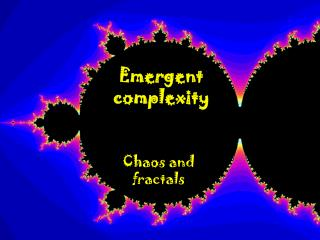DownloadDownload PresentationEmergent complexity

# Emergent complexity

Download Presentation## Emergent complexity

- - - - - - - - - - - - - - - - - - - - - - - - - - - E N D - - - - - - - - - - - - - - - - - - - - - - - - - - -
##### Presentation Transcript

1. Emergent complexity Chaos and fractals

2. c-plane Uncertain Dynamical Systems

3. Julia sets

4. Overcoming computational complexity

5. What does this have to do with complex systems? • This classic computational problem illustrates an important idea, but in an easily visualized way. • Most computational problems involve uncertain dynamical systems, from protein folding to complex network analysis. Not easily visualized. • Natural questions are typically computationally intractable, and conventional methods provide little encouragement that this can be systematically overcome.

6. Main idea e.g. the boundary moves.

7. Main idea Points near the boundary are “fragile.” Merely stating the obvious in this case. But illustrates general principle that can be exploited by the right algorithms.

8. # iterations 5 10 Points not in M. 15 20 25 30

9. # iterations 5 10 Color indicates number of iterations of simulation to show point is not in M. 15 20 25 30

10. But simulation cannot show that points are in M.

11. But simulation is fundamentally limited • Gridding is not scalable • Finite simulation inconclusive

12. Main idea It’s easy to prove that this disk is in M. Other points in M are fragile to the definition of the map. Merely stating the obvious.

13. Sufficient condition

14. Trivial to prove that these points are in Mandelbrot set.

15. Main idea The proof of this region is a bit longer. The longer the proof, the more fragile the remaining regions.

16. Main idea Proof even longer. And so on…

17. Easy to prove these points are in Mset.

18. Easy to prove these points are not in Mset.

19. Proofs get harder. (But all still “easy.”) What’s left gets more fragile.

20. Complexity • Chaos •  Fractals Emergent complexity.

21. Complexity implies fragility What matters to organized complexity.

22. Emergent complexity

23. How might this help with organized complexity and “robust yet fragile”? • Long proofs indicate a fragility. • Either a true fragility (a useful answer) or an artifact of the model (which must then be rectified) • Potentially fundamentally changes computational complexity for organized complexity • Brings back together two research areas that have been separated for decades: • Numerical analysis and ill-conditioning • Computational complexity (P, NP/coNP, undecidable)

24. Proof? New proof methods that is scalable and systematic (can be automated).

25. Breaking hard problems • SOSTOOLS proof theory and software • Nested family of (dual) proof algorithms • Each family is polynomial time • Recovers most “gold standard” algorithms as special cases, and immediately improves • No a priori polynomial bound on depth (otherwise P=NP=coNP) • Conjecture: Complexity implies fragility

26. Safety Verification and Reachability Analysis • Safety critical applications. • Exhaustive simulation is not exact. • Set propagation is computationally expensive. B(x) = 0 Find a barrier certificate B(x) Unsafe set Initial set Scalable computation using SOS machinery.

27. Hybrid, Uncertain, Stochastic Hybrid systems can be handled easily, even for systems with uncertainty: • Parametric • Memoryless • Dynamic (IQC) (Prajna, Jadbabaie – HSCC ’04) Also stochastic hybrid systems: • Use supermartingales as certificates. • Get guaranteed bound on reach • probability. (Prajna, Jadbabaie, Pappas – CDC ’04)

28. Functional requirements Unifying role of dual proofs and decomp-ositions Variable supply/demand Feedback control “Vertical” layering “Horizontal” Decompositions Physical network Components Hardware constraints

29. Main idea Think of this as a robustness problem.

30. Globally stable. How robust is stability to perturbations in c?

31. Region of convergence. How robust is stability to perturbations in c?

32. Simulation is fundamentally limited

33. Simulation is fundamentally limited

34. # iterations 5 10 15 20 25 30

35. iterations 10 20 30 40 50 60

36. iterations 10 20 -2 30 40 50 60

37. iterations 10 20 -2 30 40 50 60

38. iterations 10 20 30 40 50 60

39. iterations 10 20 30 40 50 60

40. iterations 70 120

41. iterations 60 120